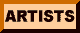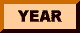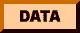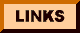Help With Chords

Listed below, by key, are chords used in these songs. I have excluded root chords (since most people know them) and also excluded most of the standard dominant seventh chords (unless a special form is called for). Chords are listed alphabetically by Root...and somewhat arbitrarily within those categories.

Chord Descriptions

As a preface to everything, let me make this clear: I use chord names to describe specific formations, which are themselves described in detail below. Those descriptions indicate which string is fretted, and where. For example, the basic E chord would be described as 022100. The numbers represent the six strings of a guitar, the one on the left being the lowest tone, theone on the right being the highest. The number means only where the string is fretted -- I make no suggestions about which finger frets it there. That's up to you.

An "x" in any string position means "Don't play this string at all." A "0" on a string means play it open -- not fretted anywhere.

Because I'm describing specific formations by using specific names, let me add that I already know some of these chord names are not technically correct -- especially diminished chords, as described below. To repeat: my chord names describe chord formations and those chord formations are described below.

A Note on Diminished Chords

As I mention on the home page of this site, my diminished chords are usually mis-named in all of these charts. Here's why: diminished chords (specifically, diminished 7th chords if you want to split hairs) are all made up of four tones, each three half-steps apart on the piano keyboard. Since they are equally spaced from each other, the actual root name for that chord depends on function in the melody. The formation I refer to as Cdim, formed as 001212 on the fretboard, is played exactly the same, whether it is functioning as a Cdim, as an E-flat diminished, as F# diminished, or as Adim.

Because it is played the same, and sounds the same, regardless of what function it is serving, I always call that formation the same thing in these charts. Why? One word: Simplicity. Why use -- and have to remember -- four different names for one formation, when it's going to sound the same regardless of what function it serves or what you call it?

The truth is, I don't know what function a given chord serves, and I don't care. I care only about the sound. If you want to know what the chord actually is, I'd suggest finding a theory book that can explain them -- and consult it any time one of my charts has a chord with the letters "dim" in it.

Within this convention, I use four primary diminished chord formations and names:
Fdim which is formed as xx0101,
Cdim which is formed as xx1212,
Gdim which is formed as xx2323, and finally, the least common,
Edim which is formed as 012020.

Very rarely, there is an alternate formation used for a couple of these -- identified in these charts by a Roman Numeral inside a parenthesis after the chord name, such as Fdim(III). When you see that, you'll begin the formation on the fret number that matches the number in the parenthesis. A chord marked Cdim(IV) will be formed as 004545. The oddball is Fdim(III) itself -- it doesn't copy the Fdim formation exactly, but it's similar: 003434.

Naming Conventions

Since computer keyboards don't have the "flat" symbol, I use a small "b" to designate "flat" so Bb means "B-flat" rather than "B-B." The "#" symbol means "sharp." The small "m" means "minor" and a large "M" means "Major."

The symbol "-" can be read as "flat" -- thus the chord Bm7-5 (you'll find it a lot in my charts...I love the way it goes to an E7) is stated as "B minor seven flat five." In general, that symbol "-" means to lower the following number by one fret, so a flat-five is the fifth moved down by one fret. But you really don't need to remember that -- just finger the chord according to the chart below and it's done for you.

You'll see the symbol "+" used a couple of ways. At the end of a chord name, like A+, it means "augmented" -- which usually implies "augmented fifth," or the fifth of the chord raised one-half step. If you see the "+" before a number, like "G+7" you can read it as "sharp" as in G sharp-seventh but you may be causing yourself unneeded confusion. While "G+7" in that sense is the same as a G major seventh (splitting hairs again), it would be awfully easy to confuse that with a "G#7" when it refers to a dominant 7th in the key of G#. All in all, it just seemed clearer to me to let the "+" symbol represent the alteration and the "#" symbol represent its more familiar key meaning and nothing else.

You will also find I use a couple of different voicings for some chords, just because a slightly different sound is achieved with each. Compare the G+7 to the GM7 below, and play them on your guitar and you'll see what I mean. Sometimes I think one "voicing" sounds better than another in the context of a given song. If so, I try to name it distinctly. (Notice chords below with the letters "alt" in them.)

Along similar lines, some of my "slash" chords which involve numbers (E7/6 for example) are not technically correct in their names. That E7/6 probably ought to be E13, or some such. But I'm since I'm using names to describe formations, it makes more sense to me to call it E7/6 than it does E13. Why? Because E13 means nothing to me; E7/6 means "start with an E7 and add in a 6th."

If you see a "slash" chord with a letter after it (you'll encounter two of them often -- G/F# and C/B) it means play a basic Major chord based on the first letter, but change the bass note to the note whose name follows the slash. Thus G/F# is a G chord with F# in the bass (fingered 220003) and C/B would be a C chord with a B bass note (played x22010).

Transposing

Even if you're a complete amateur at the process, transposing any of these charts to a key better suited to your voice is simpler than you might think. All you need do is raise or lower the chord cited by a specific number of pitches, or steps. Rather than even mess your mind with, "Did he mean half steps or whole steps or baby steps or what?" you can do what I did on just about all of these charts, once I had done the initial transcription -- that is, employ The Guitarguy's very own, home-made, handy-dandy Transposition Wheel: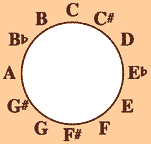Every chord, no matter how much it's been altered, begins with a letter designation for the primary name -- whether its obvious like C or A or D, or a little more abstracted like F# or Bb. If you need to go from the key of C to the key of F, for example, consult the wheel: move five steps clock-wise and you're there. Now, do the same thing for every chord your encounter, but leave the modifier the same as it already is -- for example, a Bm7-5 moves the initial "B" the same number of steps, in the same direction, to become Em7-5.

How do you know how many steps to use? Depends. How many are comfortable? If the key of C is 'way too low, skip up (or down) several steps. It's purely a matter of choice and personal comfort. I use the key of D a lot in these charts, for a couple of reasons. First, it's comfortable for me to sing in that key most of the time. Second, because I use that key a lot, I am very familiar with the chord variations that add "color" in that key, without having to think too much about how to form them.

And that, my friend, is all there is to it.

Chords used in these songs

The following are chords that I have used in constructing these my charts:

Ab=xx111x
Ab+=xx211x

A

A(V)=577655
A+=003220
A-9=002320
A6=002222
A6alt=004220
A6/7/9=002002
A7+9=032020
A7+5=003020
A7-9=002320
A7-5=001020
A7/6(V)=575675
A7/9=002000
A7/9+5=003000
A7sus4=002030
A9=002200
Ab=xx111x
Am+5=003210
Am+7=000211
Am7/6=004010
Am6=004210 or 002212
Am7 alt=002013
Am7-5=001010
AM7=002120
AM7/9=02100
AM7/6=002122
Am/F#=202210
Am7/F#=202010
Am7/G=302010
AM7=002120
AM7/9=002100 Asus4=002230

Bb7=113131
Bb9=110111
Bb=113331
BbM7=003230
BbM7 alt=003234

B+=021000
B7(II)=224242
B7/9=x2122x
B7sus4=x22202
Bm7-5=023230
Bm7=004432
Bm7sus4=004430
BM7=xx434x
Bm=224432
Bm7/E=004432
Bm9=220222

C

C/B=020010
C6=x32210
C7alt='xx2313
C7/9=032330
C9=332030
C alt=032013
Cdim(IV)=004545
Cdim=xx1212
Cm(7)=005543 or =335343
CM7/6=332200
CM7/9=332030
Cm7=005543
CM7=332000
Cm9=005545
Cm=335543
Csus4=332011

C#7=x43404
C#7/G#=4x3404
C#m7-5=045450
C#m7=xx6654
C#m7alt=xx6650
C#m=446654

D

D alt=004235
D+=000332 (also called D+5)
D6/9=000200
D6=000202
D/F#-2002xx
D6sus4=020032
D7+=000312
D7-5=000112
D7/9=000210
D7sus4=000213
D9=004230
D9alt=000230
D9sus4=002033
Dm+7=000221
Dm6=000201
Dm7-5=000111
Dm7-5=056560 (alternate formation)
DM7=000222
DM7/9=00220
Dm7sus4=000213
Dsus4=000233

Eb6=xx1313
Eb=xx1343
EbM7=xx1333

E

E+7=021100
E+=032100
E-9=023100
E6=022120 (or 042100)
E7+5=020110 (or 030100 or 032130)
E7alt=020130
E7+=000110
E7-5=012030
E7-9=023130
E7/6=020120
E7/9=020102
E7sus4=020200
E9=024100
Edim=012020
Em+5=022020
Em/F#=222000
Em6 alt=042000
Em6=022020
Emsus4=022200
Em7-5=078780
Em7/9=020002
Em7sus4=020200
Em9=024000
Esus4=022200

F6=133231
F7=131211
F7/9=131213
F7-9=034240
Fdim(III)=xx3434
Fdim=xx0101
Fm7/6=131131
Fm7/9=131113
FM7=033210

F#7-9=045340
F#7=242322
F#=244322
F#6=244342
F#m7=242222
F#m7/6=242242
F#m7-5=202210
F#malt=244225
F#m+7=244224

G

G+7=320002
G+=xx1003
G-9=320004
G/F#=220003
G/B=020030
G5=32003x
G5/7=32303x
G6=320000 or 302003
G6/9=000203
G7+5=xx1001
G7-9=056460
G7+=xx1001
G7/6=323000
G7alt=323003
G9=300203
G9=300203
Gdim-xx2323
Gm6=355353
GM7/6=324000
GM7=32400x
Gm7=353333